# Numerical simulation of Cu-water nanofluid magneto-hydro-dynamics and heat transfer in a cavity containing a circular cylinder of different size and positions

Numerical simulation of Cu-water nanofluid magneto-hydro-dynamics and heat transfer in a cavity containing a circular cylinder of different size and positions

Faculty of Engineering, Mechanical Engineering Department, Ferdowsi University of Mashhad, Iran

Faculty of Engineering, Electrical and Electronics Engineering Department, Shiraz University of Technology, Iran

Corresponding Author Email:
javareshkian@um.ac.ir
Page:
403-415
|
DOI:
https://doi.org/10.18280/ijht.350225
|
Accepted:
|
Published:
30 June 2017
| Citation

OPEN ACCESS

Abstract:

In the present study, a nanofluid filled cavity with sinusoidal temperature boundary condition, under the influence of an inclined magnetic field is investigated numerically. The LBM method is applied to simulate the Cu-Water nanofluid flow around an average temperature circular cylinder. In this study, the different braking manners of the magnetic field and the obstacle are compared for different field intensities and directions and various obstacle sizes and positions. The flow and heat transfer behavior of the nanofluid were observed for different Rayleigh numbers (103–106), Hartmann numbers (0–90), nanoparticles' volume fraction (0–6 %), magnetic field direction = (0–90o) and obstacle aspect ratios (0.05, 0.1 and 0.2) and positions (0-8). The results indicated that the influence of nanoparticles for this geometry and boundary condition is highly dependent on the Rayleigh and Hartmann numbers. Also, it was shown that for lower Ra numbers, the obstacle with an aspect ratio of 0.1 presents better heat transfer rate; while for higher Ra numbers the obstacle size is much less important than its position.

Keywords:

circular obstacle, nanoparticles' volume fraction, magnetic field intensity, direction

1. Introduction

Choi and Eastman  were the first to refer a two-component fluid consisting of medium liquid and solid nanoparticles with the word Nanofluid. Their pioneering study in 1995 opened an endless era of research, innovations, and modifications on the subject. Due to higher thermal susceptibility, these newly born colloidal fluids proved to be an important asset for heat transfer systems. Comparing to pure coolants, nanofluids possess higher thermal conductivity and unlike microfluids, they were much more stable. So they could raise the heat transfer rate of the system without any critical concern of aggregation or sedimentation in the circulation system components. This was a promising situation for many who were looking for more capable coolants [2-7].

Khanafar et al.  tried to simulate numerically the flow and heat transfer of Cu-Water nanofluid using finite volume approach. In their work, four different methods for nanofluid treatment were examined and the results were compared to the experimental data. A numerical investigation on natural convection cooling of an oscillating heat source fitted on the left wall of an enclosure was performed by Ghasemi and Aminossadati . Cu, Al2O3, and TiO2 nanoparticles dispersed in water were used as working fluids. It was concluded that the inclusion of dispersed nanoparticles into the base fluid improves its thermal conductivity and enhances the heat removal from the heat source. The most effective nanoparticle was reported to be Cu in the heat removal process, followed by Al2O3 and TiO2 respectively. Kalteh et al.  studied the steady laminar mixed convection of water-based nanofluid flow in a lid-driven square cavity with a triangular heat source. It was found that suspending the nanoparticles in pure fluid leads to a significant heat transfer increase.

Another uprising field of interest among researchers is Magnetohydrodynamics. MHD is the study of the dynamics of electrically conducting fluids. Examples of such fluids include plasmas, liquid metals, salt water or electrolytes, and of course, nanofluids. The field of MHD was initiated by Hannes Alfven , for which he received the Nobel Prize in physics in 1970. The fundamental concept behind MHD is that magnetic fields can induce currents in a moving conductive fluid, which in turn creates forces on the fluid and changes the magnetic field itself. The wide occurrence of Magnetohydrodynamics in many industrial cases like crystal growth, metal casting, fusion reactors and geothermal energy extractions makes it undeniable [12-16].

Al-Nimr  obtained analytical solutions for MHD fully developed upward (heating) or downward (cooling) natural convection in open-ended porous annuli, in 1995. Later on, Al-Nimr and Hader  modified their solution for more general thermal boundary conditions. Oztop et al.  made a numerical study on the MHD mixed convection in a lid-driven cavity with corner heater. They applied a finite volume technique to observe the fluid flow and temperature fields under different Grashof and Hartmann numbers. They have taken the Joule effect into account in their study. Ahrar et al.  simulated the MHD convection of air in a lid-driven cavity with heated walls. They studied the influence of Hartmann number and magnetic field direction on the convection heat transfer rate and proposed the optimum situations for heat transfer rate.

When nanofluids were applied in MHD researchers' projects, not only because of their raised electrical conductivity but also for their coexistence with magnetic situations in many of their applications, they were targeted by many MHD researchers. When unpredictable behaviors of nanofluids wed the complexity of MHD, one steps into a dark and unknown area of science, which attracts a large number of scholars. Different solution techniques and test cases with the specific concept about MHD of nanofluids have been considered in the open literature so far [21-25] and there is always room for more.

In 2011, Ghasemi et al.  also used FV method to investigate the Al2O3-Water nanofluid flow characteristics under the influence of a magnetic field source. They proclaimed that the heat transfer rate increases with an increase of the Rayleigh number but it decreases with the increment of Hartmann number. They also reported that for certain Ha and Ra numbers there could be situations that adding Al2O3 nanoparticles would decrease the Nu number. Nemati et al.  used LBM method to study the Magnetic field effects on natural convection flow of different nanofluids in a rectangular cavity. They found that the average Nusselt number increases for nanofluid when increasing the solid volume fraction, while in the presence of a strong magnetic field, this effect decreases.

More recent studies on nanofluids' flow, deal with: different cylinders and geometries [28, 29], different magnetic field conditions , Hybrid nanofluids [31-33] and different solution techniques [34, 35]. Although, Hybrid nanofluids seem to have a great future in front of them but currently, curved boundaries (different geometries), due to their wide occurrences in the heat transfer industries and the verity of simulation approaches, are very popular among other novel contributions [36, 37].

It is well known that addition of nanoparticles [38, 39], magnetic field intensity [38-40] and obstacle  lead to a decrease in the stream function and heat transfer rate, so in this study, we aim to define and observe the combined effect of all above-mentioned features. On the other hand, nanoparticles higher conductivity, and obstacle temperature gradient are meant to increase the heat transfer rate. So, it is tried to separate facts from fancies, by simulation of a benchmark of more than 1300 test cases including a wide range of Ha (0–90) and for magnetic field angles $\gamma=0^{\circ}$ to 90 and phase deviation of $\theta=90^{\circ}$. Evidently, Cu nanoparticle with $K_{s}=400$ is considered as one of the best nanoparticles for heat transfer [38, 42]. So in order to increase heat transfer rate, in the present work, this nanoparticle was chosen to compare the triple effects of adding nanoparticles to the fluid, changing the magnetic field intensity and direction and the obstacle aspect ratios and positions for this nonlinear boundary condition.

2. Problem Statement and Formulation

2.1 The classical form of formulation

The continuity, momentum and energy equations (1-4) for nanofluid MHD flow in dimensional form can be written as follows:

$\frac{\partial u}{\partial x}+\frac{\partial v}{\partial y}=0$    (1)

$\rho_{n f}\left(u \frac{\partial u}{\partial x}+v \frac{\partial u}{\partial y}\right)=-\frac{\partial P}{\partial x}+\mu_{n f}\left(\frac{\partial^{2} u}{\partial x^{2}}+\frac{\partial^{2} u}{\partial y^{2}}\right)+F_{x}$   (2)

$\rho_{n f}\left(u \frac{\partial v}{\partial x}+v \frac{\partial v}{\partial y}\right)=-\frac{\partial P}{\partial y}+\mu_{n f}\left(\frac{\partial^{2} v}{\partial x^{2}}+\frac{\partial^{2} v}{\partial y^{2}}\right)+F_{y}$   (3)

$u \frac{\partial T}{\partial x}+v \frac{\partial T}{\partial y}=\alpha_{n f}\left(\frac{\partial^{2} T}{\partial x^{2}}+\frac{\partial^{2} T}{\partial y^{2}}\right)$     (4)

where Fx and Fy are the total body forces in x and y directions respectively and can be defined as:

$F_{x}=\frac{H a^{2} \mu_{n f}}{L^{2}}\left(v \sin \gamma \cos \gamma-u \sin ^{2} \gamma\right)$      (5)

$F_{y}=\frac{H a^{2} \mu_{n f}}{L^{2}}\left(u \sin \gamma \cos \gamma-v \cos ^{2} \gamma\right)+\rho_{n f} g \beta_{n f}\left(T-T_{c}\right)$  (6)

In the above equations $H a=L B \sqrt{\sigma_{n f} / \mu_{n f}}$ is the Hartmann number in which $\sigma$ is electrical conductivity, B is the magnitude of the magnetic field, L is the length of the cavity and $\gamma$ is the direction of the magnetic field.

2.2 Simulation of MHD with lattice Boltzmann method

2.2.1 Brief introduction to LBM

The LBM method with standard two-dimensional, nine velocities (D2Q9) system for both temperature and flow field is applied in this study. For the sake of completeness, a brief review of Lattice Boltzmann Method is included. The discretized LBM equations with external force in nine directions can be written as:

$f_{i}\left(x+c_{i} \Delta t, t+\Delta t\right)-f_{i}(x, t)=-\frac{1}{\tau_{\nu}}\left[f_{i}(x, t)-f_{i}^{e q}(x, t)\right]+\Delta t F$   (7)

$g_{i}\left(x+c_{i} \Delta t, t+\Delta t\right)-g_{i}(x, t)=-\frac{1}{\tau_{\alpha}}\left[g_{i}(x, t)-g_{i}^{e q}(x, t)\right]$     (8)

Equations (7) and (8) are used to solve the flow and temperature fields, respectively. Eq. (7) recovers the continuity and momentum equations (1–3), where the total body forces were considered by the external force of equation (7). Eq. (8) describes the evaluation of the internal energy and leads to Eq. (4). In the above equations $f_{i}^{e q}$ and $g_{i}^{e q}$ are the equilibrium distribution functions for flow and temperature field, respectively, and can be calculated as follows:

$f_{i}^{e q}=\omega_{i} \rho\left[1+\frac{c_{i} u}{c_{S}^{2}}+\frac{1}{2} \frac{\left(c_{i} u\right)^{2}}{c_{S}^{4}}-\frac{1}{2} \frac{u . u}{c_{S}^{2}}\right]$      (9)

$g_{i}^{e q}=\omega_{i} T\left[1+\frac{c_{i} u}{c_{S}^{2}}+\frac{1}{2} \frac{\left(c_{i} \cdot u\right)^{2}}{c_{S}^{4}}-\frac{1}{2} \frac{u . u}{c_{S}^{2}}\right]$           (10)

where $C_{s}$ is the lattice speed of sound which is equal to $c_{s}=c / \sqrt{3}$ and the discrete velocities, or D2Q9 are also defined as:

$c_{i}=\left\{\begin{array}{ll}{0} & {\text { for } i=0} \\ {c \cdot\left[\cos \left(\frac{i \pi}{2}-\frac{\pi}{2}\right), \sin \left(\frac{i \pi}{2}-\frac{\pi}{2}\right)\right]} & {\text { for } i=1-4} \\ {c \sqrt{2} \cdot\left[\cos \left(\frac{i \pi}{2}-\frac{9 \pi}{4}\right), \sin \left(\frac{i \pi}{2}-\frac{9 \pi}{4}\right)\right]} & {\text { for } i=5-8}\end{array}\right.$   (11)

In the above equations, c is equal to $\Delta x / \Delta t$, with $\Delta x$ and $\Delta t$ being the lattice space and lattice time step, respectively. The weighing factors for the D2Q9 model are obtained as:

$\omega_{i}=\left\{\begin{array}{ll}{4 / 9} & {\text { for } i=0} \\ {1 / 9} & {\text { for } i=1-4} \\ {1 / 36} & {\text { for } i=5-8}\end{array}\right.$          (12)

The kinematic viscosity $v$ and the thermal diffusivity $\alpha$ are then related to the relaxation times by equations (13) and (14):

$v=\left[\tau_{v}-\frac{1}{2}\right] c_{s}^{2} \Delta t$      (13)

$\alpha=\left[\tau_{\alpha}-\frac{1}{2}\right] c_{s}^{2} \Delta t$    (14)

2.2.2 Applying body forces in LBM

In order to apply the effect of magnetic field and gravitational forces (body forces), a force term F was added to the density distribution function equations. To obtain F all variables must become dimensionless with the lattice units. So, the external force appears for LBM as follows :

In the above equations, M is the number of lattices in the specific length L direction, and the total body force term F can be produced from the summation of (17) and (18).

$F=F_{x}+F_{y}$       (15)

$F_{i}=\frac{\omega_{i} F \cdot c_{i}}{c_{s}^{2}}$       (16)

$F_{i x}=3 \omega_{i} \rho\left[\frac{H a^{2} v}{M^{2}}\left(v \sin \theta \cos \theta-u \sin ^{2} \theta\right)\right]$       (17)

$F_{t y}=3 \omega_{i} \rho\left[\left(g \beta\left(T-T_{c}\right)\right)+\frac{H a^{2} v}{M^{2}}\left(u \sin \theta \cos \theta-v \cos ^{2} \theta\right)\right]$     (18)

2.2.3 Curved boundary treatment for flow field

For treating velocity and temperature fields with curved boundaries, the method proposed by Yan and Zu  has been used. An arbitrary curved wall separating the solid region from the fluid is shown in Fig. 1. The link between the fluid node xf and the wall node xw intersects the physical boundary at xb. The fraction of the intersected link in the fluid region is $\Delta=\left|x_{f}-x_{w}\right| /\left|x_{f}-x_{b}\right|$ . To calculate the post-collision distribution function  $\tilde{f}_{\overline{k}}\left(x_{b}, t\right)$ based on the surrounding node information, we can write:

$\tilde{f}_{\overline{k}}\left(x_{b}, t\right)=(1-\chi) \tilde{f}_{k}\left(x_{f}, t\right)+\chi f_{k}^{*}\left(x_{b}, t\right)+2 w_{k} \rho \frac{3}{c^{2}} e_{\overline{k}} \cdot u_{w}$     (19)

In this equation, we have:

$f_{k}^{*}\left(x_{b}, t\right)=f_{k}^{e q}\left(x_{f}, t\right)+w_{k} \rho\left(x_{f}, t\right) \frac{3}{c^{2}} e_{k} \cdot\left(u_{b f}-u_{f}\right)$

$u_{b f}=u_{f j}=u\left(x_{f f}, t\right), \quad \chi=\frac{(2 \Delta-1)}{\tau-2}, \quad$ if $\quad 0 \leq \Delta \leq 0.5$                        (20)

$u_{b f}=\frac{1}{2 \Delta}(2 \Delta-3) u_{f}+\frac{3}{2 \Delta} u_{w}, \quad \chi=\frac{(2 \Delta-1)}{\tau-1 / 2}, \quad$ if $\quad 0.5 \leq \Delta \leq 1$

where $\vec{e}_{\overline{k}}=-\vec{e}_{k}$ , $u_{f}$ is the fluid velocity near the wall, $u_{w}$ is the velocity of the solid wall, and $u_{b f}$ is an imaginary velocity for interpolations.

## 1.png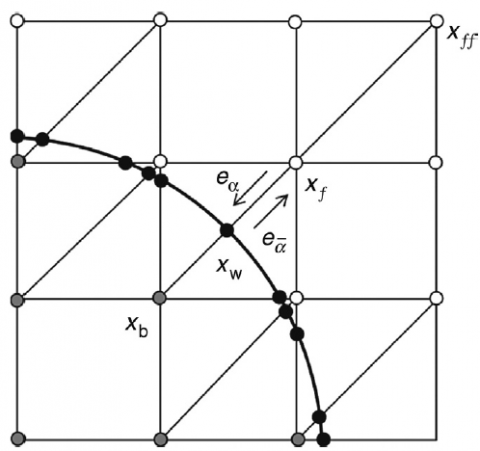Figure 1. Discrete velocity set of two-dimensional nine-velocity (D2Q9) model and curved boundary

2.2.4 Curved boundary treatment for temperature field

Following the work of Yan and Zu , the temperature distribution function can be defined with its equilibrium and non-equilibrium parts as:

$\tilde{g}_{\overline{k}}\left(x_{b}, t+\Delta t\right)=g_{\overline{k}}^{e q}\left(x_{b}, t\right)+\left(1-\frac{1}{\tau_{s}}\right) g_{\overline{k}}^{n e q}\left(x_{b}, t\right)$        (21)

Obviously, both $g_{\overline{k}}^{e q}\left(x_{b}, t\right)$ and $g_{\overline{k}}^{n e q}\left(x_{b}, t\right)$ are needed to calculate the value of $\tilde{g}_{\overline{k}}\left(x_{b}, t+\Delta t\right)$ . In above equation, the equilibrium part is defined as:

$g_{\overline{k}}^{e q}\left(x_{b}, t\right)=w_{\overline{k}} T_{b}^{*}\left(1+\frac{3}{c^{2}} e_{\overline{k}} \cdot u_{b}^{*}\right)$      (22)

In which $T_{b}^{*}$ and $u_{b}^{*}$ can be defined as:

$T_{b}^{*}=T_{b 1}, \quad u_{b}^{*}=u_{b 1}, \quad$ if $\Delta \geq 0.75$

$T_{b}^{*}=T_{b 1}+(1-\Delta) T_{b 2}, u_{b}^{*}=u_{b 1}+(1-\Delta) u_{b 2},$ if $\Delta \leq 0.75$       (23)

where,

$T_{b 1}=\left[T_{w}+(\Delta-1) T_{f}\right] / \Delta, \quad u_{b 1}=\left[u_{w}+(\Delta-1) u_{f}\right] / \Delta$

$T_{b 2}=\left[2 T_{w}+(\Delta-1) T_{f f}\right] /(1+\Delta), \quad u_{b 2}=\left[2 u_{w}+(\Delta-1) u_{f f}\right] /(1+\Delta)$      (24)

And finally, the non-equilibrium part may be computed from:

$g_{k}^{n e q}\left(x_{b}, t\right)=\Delta g_{k}^{n e q}\left(x_{f}, t\right)+(1-\Delta) g_{k}^{n e q}\left(x_{f f}, t\right)$        (25)

2.2.5 Non-dimensional parameters

For fixed Rayleigh, Mach and Prandtl numbers the kinematic viscosity can be calculated from the following equation:

$v_{f}=M \cdot \operatorname{Ma\cdot c}_{s} \sqrt{\frac{\operatorname{Pr}}{R a}}$    (26)

Also, the thermal diffusivity can be defined from the Prandtl number as:

$\operatorname{Pr}=\frac{v_{f}}{\alpha_{f}}$   (27)

In the above correlations, the Rayleigh number is defined as $R a=g \beta_{f} M^{3}\left(T_{h}-T_{c}\right) / v_{f} \alpha_{f}$ and in order to avoid any compressibility errors, the Ma number is kept at 0.1. Evidently, the Nusselt number is one of the most important dimensionless parameters in the description of the convective heat transfer. In this paper, the local Nusselt number of each wall, the average Nu on the heating part of both walls, $N u^{*}(\phi)$ and $\widehat{N u}(H a, \theta)$ are calculated from the following equations:

## 2.png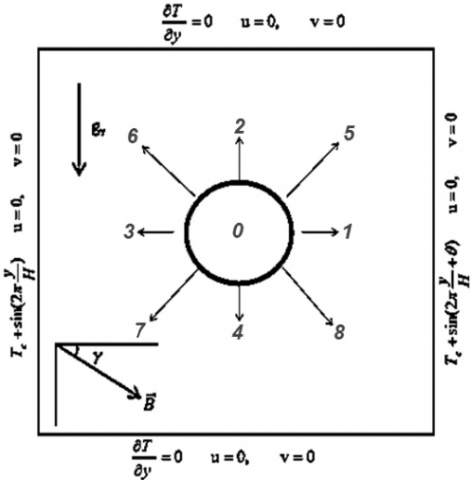Figure 2. The schematic outline of the geometry including the boundary conditions

$N u(y)=-\frac{K_{n f}}{K_{f}} \frac{M}{T_{h}-T_{c}} \frac{\partial T}{\partial n}$       (28)

$\overline{N u}=\frac{1}{H} \int_{\text {Heating half}} N u_{l e f t \text { wall }} d y+\frac{1}{H} \int_{\text {Heating half}} N u_{\text {right wall}}, d y$    (29)

$N u^{*}(\varphi)=\frac{\overline{N u}(\varphi)}{\overline{N u}(\varphi=0)}$     (30)

$\widehat{N u}(H a, \theta)=\frac{\overline{N u}(c y l, \text {pre.})}{\overline{N u}(c y l . \text { abs. })}$     (31)

Here, $N u^{*}(\phi)$ refers to the ratio of the average Nu with the nanoparticles volume fraction of $\phi$ to the average Nu number of the pure fluid.

2.3 Modeling of nanofluid

In this simulation, the dynamical similarity depends on the dimensionless parameters of Rayleigh (Ra), Prandtl (Pr) and Hartmann numbers (Ha). Due to the assumption of the nanofluid as a pure fluid, the mixture qualities are to be calculated and applied in these dimensionless parameters. The thermophysical properties of the nanofluid are fixed during the iteration process, except for the density which is obtained from Boussinesq assumption.

The density $(\rho)$, the specific heat $\left(C_{p}\right)$ and the thermal expansion coefficient $(\beta)$ of the nanofluid are calculated from below :

$\rho_{n f}=(1-\phi) \rho_{f}+\phi \rho_{s}$      (32)

$\left(\rho C_{p}\right)_{n f}=(1-\phi)\left(\rho C_{p}\right)_{f}+\phi\left(\rho C_{p}\right)_{s}$      (33)

$(\rho \beta)_{n f}=(1-\phi)(\rho \beta)_{f}+\phi(\rho \beta)_{s}$    (34)

Also, according to Brinkman model the viscosity of a mixture containing a dilute suspension of small rigid spherical particles can be obtained from :

$\mu_{n f}=\frac{\mu_{f}}{(1-\phi)^{2.5}}$      (35)

And the effective thermal conductivity of the mixture can be approximated by the Maxwell-Garnetts (MG) model as :

$\frac{K_{n f}}{K_{f}}=\frac{K_{s}+2 K_{f}+2\left(K_{f}-K_{s}\right)}{K_{s}+2 K_{f}-\phi\left(K_{f}-K_{s}\right)}$    (36)

Also, it is worth mentioning that the MG model is only restricted to spherical nanoparticles and does not take into account the shape of nanoparticles. Finally, the electrical conductivity $\sigma$ of the nanofluid is calculated from the Maxwell model :

$\frac{\sigma_{n f}}{\sigma_{f}}=\left[1+3 \phi\left(\frac{\sigma_{s}}{\sigma_{f}}-1\right)\right] /\left[\left(\frac{\sigma_{s}}{\sigma_{f}}+2\right)-\phi\left(\frac{\sigma_{s}}{\sigma_{f}}-1\right)\right]$     (37)

With $\varphi$ being the solid volume fraction and subscripts nf, f and s referring to the nanofluid, carrier fluid, and the nanoparticles, respectively. The thermophysical properties of water and the nanoparticles are presented in table 1.

Table 1. Thermophysical properties of nanoparticles and the carrier fluid

 $\mu(k g / m s)$ $\rho\left(k g / m^{3}\right)$ $C_{p}(J / k g K)$ $K(W / m K)$ $\beta(1 / K) \times 10^{-5}$ $\sigma(\Omega \cdot m)^{-1}$ water 0.00089 997.1 4179 0.613 21 0.05 Cu NPs --- 8954 383 400 1.67 $596 \times 10^{5}$
3. Numerical Approach and Validation

3.1 Geometry and boundary conditions

Fig. 1 shows a schematic outline of the enclosure filled with Cu-water nanofluid. The cavity is bounded by two adiabatic walls (south and north) and two sinusoidal varying temperature walls (west and east). The phase deviation between these walls is equal to $\pi / 2$ . Also, there is a circular obstacle with the temperature of Tc and an aspect ratio of Ar located in 9 different positions in the cavity.  In this study, it is

assumed that the nanofluid is Newtonian, the induced magnetic field produced by the motion of an electrically conducting fluid is negligible compared to the applied magnetic field, the nanoparticles are in thermal equilibrium with water and they flow with the same velocity due to assumption of no-slip velocity mechanism between the base fluid and the nanoparticles. The flow regime is also considered to be steady, two-dimensional, incompressible and laminar. Moreover, the effects of Joule heating, viscous dissipation, and Radiation heat transfer are neglected in the current study.

3.2 Code validation and mesh independence

## 3.png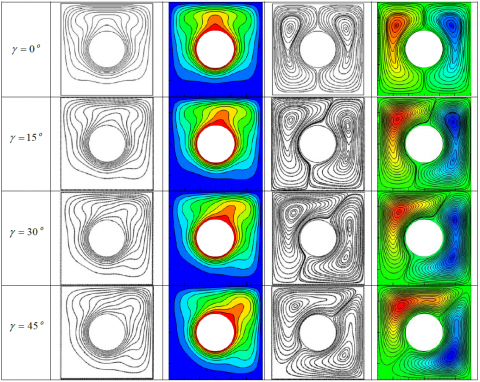Figure 3. The streamlines and isotherms for the present study(left) and Park et al.  (right) for

Ra=105, Pr=6.2 and Ar=0.2 for four different cavity angles

In the present study, an in-house SRT lattice Boltzmann code was developed in Fortran to simulate the flow and temperature fields in the mentioned cavity. The numerical results were validated with 4 different published data. At first, the natural convection with the Boussinesq assumption in the presence of a circular blockage was tested against the study of Park et al.  for Ra=105, Ar=0.1. The results for the streamlines and isotherms are shown in Figure 3. Then, the reliability of the code in the simulation of nanofluids' flow was investigated. The predicted data for Gr=105 and $\phi=10 \%$ for a uniform temperature boundary condition with Cu nanoparticles were compared to Khanafar et al.  and Jahanshahi et al.  results. Fig 4 presents the dimensionless temperature distribution along the horizontal middle section line of the cavity.  Afterward, in order to show the accuracy of the simulations under the influence of a magnetic field, the average Nusselt numbers of the present study and Ghasemi et al.  at Ra=105, for 3 different Ha numbers of: 0, 30 and 60 and volume fractions of: 0, 4 and 6 % are listed in table 2. The nanoparticles of this case are Al2O3 and the boundary conditions are uniform temperature BCs. The results in all cases showed perfect match and proved this developed code, to be a quality tool in the simulations of MHD natural convection for nanofluids. Also to show the simulations are mesh-independent, the results of the average Nusselt number for the highest Hartmann (Ha=90) and highest aspect ratio (Ar=0.2) in this study and for Ra=106 and $\phi=6 \%$ are presented in fig 5 for 6 different meshes of 40*40, 60*60, 80*80, 100*100, 120*120, 140*140. As can be seen, there is a negligible difference between the results of the meshes of 120*120 and 140*140. So in this study 120*120 mesh was chosen for the rest of the simulations.

Table 2. Comparison of the results of (Nu) for the present study and Ghasemi et al. 

 $\phi=0.00$(present study) $\phi=0.00$(Ghasemi et al.) $\phi=0.04$(present study) $\phi=0.04$(Ghasemi et al.) $\phi=0.06$ (present study) $\phi=0.06$(Ghasemi et al.) Ha=0 4.721503 4.738 4.88497 4.896 4.959111 4.968 Ha=30 3.142508 3.150 3.111831 3.124 3.090939 3.108 Ha=60 1.864826 1.851 1.812983 1.815 1.796061 1.806

## 4.png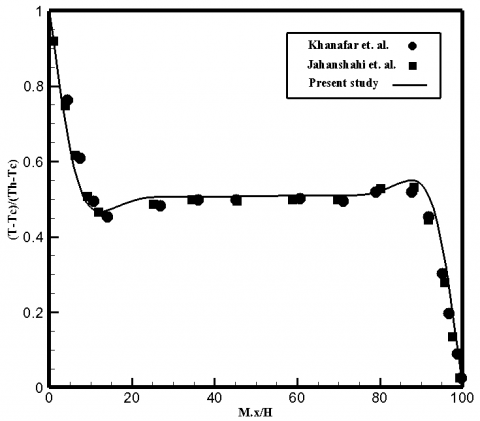Figure 4. Validation of the results for nanofluid flow at Gr=105, Pr=6.2 and $\phi=10 \%$ for the present study and Khanafer et al.  and Jahanshahi et al. 

## 5.pngFigure 5. The results of Mesh independence investigation for 6 uniform meshes

4. Results and Discussion

Figures 6-a and 6-b present the effects of magnetic field intensity and obstacle size augmentation on flow streamlines and isotherms, respectively. It is expected that both of these increments result in a reduction of the flow velocity and stream function and consequently, the average Nusselt number of the walls and the heat transfer rate would decrease. But as can be seen, the braking effect of each phenomenon is different. In the case of Ha increase, one can see that the main vortices do not change significantly and they just tend to develop in the magnetic field direction (here $\gamma=0$ ) while their velocity components are weakening due to MHD forces.

The mentioned loss of the velocity magnitude can be reasoned like this: As the streamlines try to align along the magnetic field lines, they dissipate some of the flow energy and thus the flow velocity and stream functions reduce. This can be seen on the isotherms expansion as the magnetic field intensity increases. On the other hand, the growth of obstacle aspect

ratio also influences the flow energy, directly. The obstacle retards the flow streamlines around it and sometimes it will cause a break in the main vortices. The new smaller vortices do not possess their parent vortex energy and eventually, they will lead to a decrease in the velocity field and heat transfer rate of the walls. So, the obstacle does not necessarily decrease the heat transfer rate and it depends on other flow characteristics.

Figures 7-a and 7-b demonstrate the flow streamlines and isotherms at Ra=105, Ha=60 and $\gamma=45^{\circ}$ for 9 different cylinder positions. The mentioned situation was picked from them all, because of its impressive behavior and significant results. However, the overall outcomes of other magnetic situations are presented in the next coming graphs and figures. As can be seen, the obstacle can lead the main vortex to the opposite site of the cavity in most cases. This can enhance the flow velocity on these walls and eventually, would increase the local Nusselt number of that wall. Also, when the obstacle is close to a hot part of the wall, it can improve the heattransfer due to its temperature gradient with the wall. Thus, it compacts the hot isotherms on the wall and increases the local Nu number. These two phenomena can define the overall behavior of the obstacle in almost every regime of flow.

But when the obstacle is located in the middle sites of the cavity (positions: 0, 2 and 4) it will change the flow differently. On sites 0 and 4 the main vortex of the flow breaks down into two weaker vortices and as a result, flow velocity on the walls would decrease along with the Nu number. Moreover, the obstacle is not located in the neighborhood of any hot wall, so this phenomenon is not working on these sites either. Consequently, the heat transfer of both walls would decrease drastically and they would show the least heat transfer rate among all other sites. According to this discussion, one can expect that the best heat transfer rate would be observed on sites 5 and 7 for they have both above-mentioned phenomena at their maximum influence on the system.

## 6a.png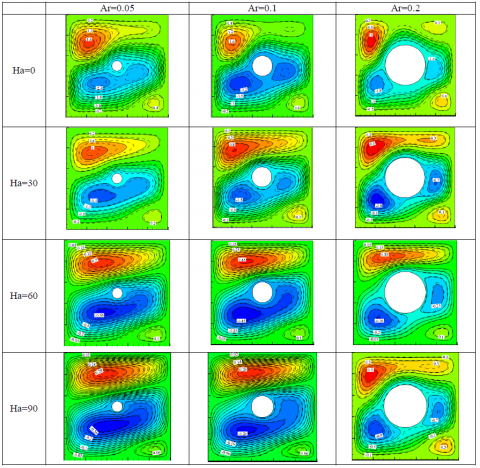Figure 6-a. Streamlines of the flow at Ra=105 and $\gamma=0$ for $H a=0,30,60,90$ and $A r=0.05,0.1,0.2$ for $\phi=0$ (solid lines) and $\phi=6 \%$ (dashed lines)

## 6b1.png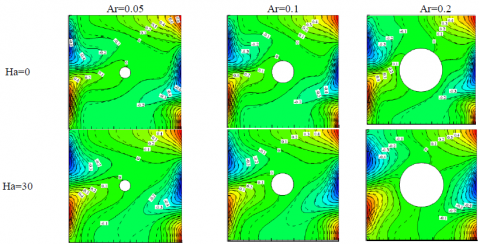## 6b2.png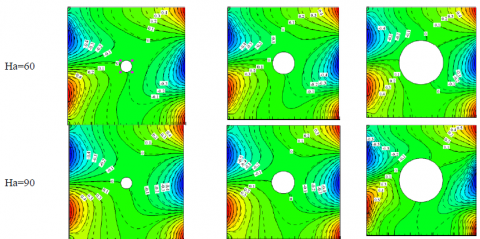Figure 6-b. Isotherms of the flow at Ra=105¬¬ and for and for (solid lines) and (dashed lines)

## 7a.pngFigure 7-a. Streamlines of the flow at Ra=105 and $H a=60$ for $\gamma=45$ and $A r=0.1$ for $\varphi=0$ (solid lines) and $\varphi=6 \%$ (dashed lines) for 9 cylinder positions

## 7b1.png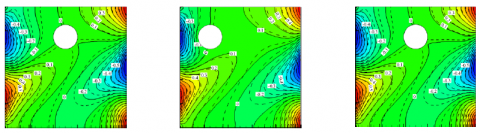## 7b2.png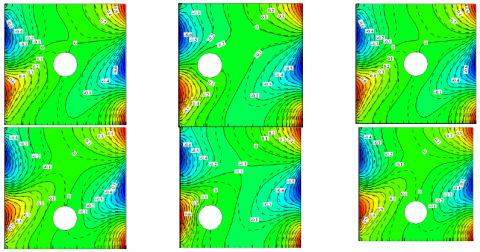Figure 7-b. Isotherms of the flow at Ra=105¬¬ and for and for (solid lines) and (dashed lines) for 9 cylinder positions

## 8.pngFigure 8. Nu* versus Ra number for different aspect ratios: 0.05, 0.1 and 0.2

In figure 8, the Nu* is plotted against the Ra number for three different obstacle aspect ratios. As can be seen, the Ar=0.1 shows the highest oscillating manners. In which the Nu* is in its maximum positions for the low Ra cases (Ra<104) and again it reduces to the minimum for higher Ra cases (Ra>104). For the other aspect ratios, the behavior of Nu* for an increment of Ra is almost smooth and basically, with an increase in Ra the obstacle in position 0 can cause a drastic decrease in the Nu* in the absence of the magnetic field. This is due to the stream function reduction caused by the obstacle. This behavior is seen more pronounced in higher Ra numbers because in lower Ra numbers the dominant heat transfer regime is the conduction and the flow velocity on the walls is relatively low. On the other hand, in higher Ra numbers the dominant heat transfer regime is convection and when the blockage is located in the center of the cavity, it can change the flow velocity on the walls significantly.

Other valuable feature of this graph, tells us that with an increase of the blockage size (aspect ratio), the heat transfer rate does not necessarily decrease. As was mentioned before, this obstacle is cold (Tc) and when it comes near to hot walls, due to the temperature gradient it can enhance the Nu number. This phenomenon has caused the Nu* to be almost the same for Ar=0.2 and Ar=0.05. Also, this is the same reason that the Nu* changes manner for Ar=0.1. In lower Ra numbers, the obstacle may be looked as a local heat sink but in higher Ra numbers, the obstacle mostly acts like a blockage.

Figure 9 presents the mean Nusselt number of the walls of the cavity in the presence of the cylinder, normalized by the Nusselt number of the case of its absence ( $\widehat{N u}$ ). In all graphs, $\widehat{N u}$ is raising with the particles' volume fraction augmentation, from which one can deduce that in the case of obstacle presence the nanoparticles addition always would be helpful. Many authors [39,42] believe that this assumption is not always reliable in the absence of the obstacle. Also, we can see, there is one minimum point on the $\varphi=6 \%$ cases and 1 relative maximum point on $\varphi=0 \%$ cases. Which means at the magnetic inclination angles around 45o, the circulation is in its best situation so the normalized effect of obstacle presence is minimized and the overall effect of it on the pure fluid is shown as a maximum point for its better circulation.

According to this figure, Positions 7 and 1 indicate the best overall effect of obstacle presence on the flow regime and other positions do not indicate any significant preferences. In its best situation (obstacle position and magnetic field direction), the addition of the obstacle can cause a maximum of 30 % increase of the normalized Nusselt number. Almost in all cases for $\varphi=0 \%$ the presence of the obstacle reduces the heat transfer rate. On the contrary again in all cases, the obstacle can improve the normalized Nu for $\varphi=6 \%$ and in the case of $\varphi=3 \%$ the obstacle heat transfer enhancing ability is squared by its reduction influence on the stream function.

## 9.png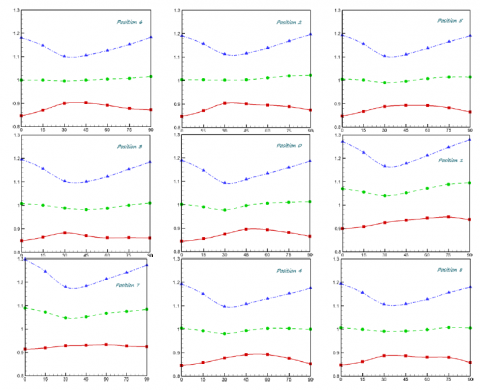Figure 9. $\widehat{N u}$ for Ra=105, Ha=90 and Ar=0.1 versus different magnetic field directions: $\varphi=0 \%$ (solid line), $\varphi=3 \%$ (dashed line), $\varphi=6 \%$ (dash-dot line).

## 10.png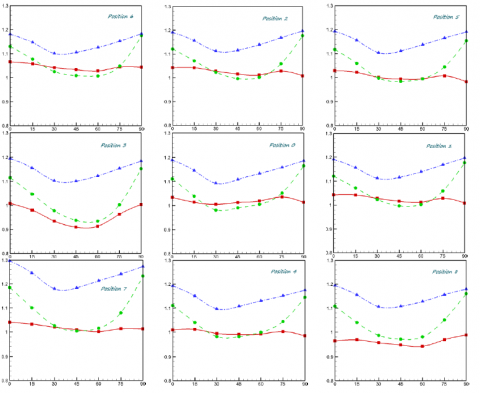Figure 10. $\widehat{N u}$ for Ra=105, $\varphi=6 \%$ and Ar=0.1 versus different magnetic field directions: Ha=30 (solid line),  Ha=60 (dashed line),  Ha=90 (dash-dot line).

Figure 10 demonstrates the variation of the normalized Nusselt number $\widehat{N u}$ with respect to the variation of the magnetic field intensity and direction for the 9 obstacle positions. As can be seen, three Ha numbers of 30, 60 and 90 and 7 magnetic field directions $\gamma$ s are chosen to describe the mentioned phenomenon. It is obvious that almost in all cases the highest influence of the obstacle is observed for Ha=90. This is well predicted because the flow regime has lower stream function values and thus lower flow rates on the walls in higher Ha numbers. However, it is interesting to see that this fact is not valid for Ha=60 and Ha=30. For lower Ha numbers, the magnetic forces are weaker so the flow is not totally under the influences of these forces. In such cases, the position of the obstacle becomes more valuable to the flow regime than the magnetic forces. That is why in some cases at $\gamma$ s around 45o the $\widehat{N u}$ for Ha=30 exceeds than the ones for Ha=60.

This magnetic field angle is the magnetic situation that provides the best circulation, so adding the obstacle with its reduction of the fluid velocity on the walls, may not be a very effective idea to improve the heat transfer rate. Also, it is seen that for two obstacle positions (namely 5 and 8) the above-mentioned increase of Ha=30 is not observed but with a more precise observation, we can see that in these points the overall $\widehat{N u}$ is reduced. Thus it can be concluded that these positions can not provide a fine situation for the fluid flow nor for the heat transfer. These positions showed the same results in figure 9.

5. Conclusion

In the present study, the influence of magnetic field intensity and direction on a nanofluid-filled closed cavity with sinusoidal temperature boundary condition was investigated numerically in the presence of a circular cylinder. Three different aspect ratios (0.05, 0.1 and 0.2) and 9 different locations were chosen for the cylinder to explain its effects on the flow and heat transfer rate under the influence of a magnetic field. The main findings of the numerical simulations are as follows:

1. It is well known that the blockage and the magnetic field both has a reducing effect on the stream function and heat transfer rate, but in this study, one
2. can observe that the behaviors of these reductions are not the same for both phenomena.
3. The obstacle has an average temperature of Tc, but from heat transfer viewpoint, it can cause 2 different issues: firstly, it will decrease the stream function and Nusselt number of the walls and secondly, it can improve the heat transfer rate by its temperature gradient with the walls of the cavity.
4. The effect of the addition of Cu nanoparticles is more pronounced in lower Ra numbers, but the cylinder size is an important issue to be taken into account. As an example, the cylinder with Ar=0.1 demonstrates the best heat transfer rate in lower Ra numbers.
5. Positions 7 and 1 indicate the best overall effect of obstacle presence on the flow regime and other positions do not indicate any significant preferences. In its best situation (obstacle position and magnetic field direction), the addition of the obstacle can cause a maximum of 30 % increase of the normalized Nusselt number.
6. The highest influence of the obstacle is observed for Ha=90 among other magnetic field intensities due to the lower stream function values. However, this fact is not proved to be valid for Ha=60 and Ha=30 in which the magnetic forces are weaker, that is why in $\gamma$ s around 45o the $\widehat{N u}$ for Ha=30 exceeds than the ones for Ha=60
Acknowledgment

This work was supported in part by Ferdowsi University of Mashhad’s Research Council, under grant #2/38801 (dated 26-10-2015) on Static Fine Job.

References

 Choi S., Eastman J.A. (1995). Enhancing thermal conductivity of fluids with nanoparticles, ASME-Publications-FED, pp. 23199-106.

 Wen D.S., Lin G.P., Vafaei S., Zhang K. (2009). Review of nanofluids for heat transfer applications, Particuology, Vol. 7, No. 2, pp. 141-150.

 Wang X.Q., Arun S.M. (2008). A review on nanofluids-part i: theoretical and numerical investigations, Brazilian Journal of Chemical Engineering, Vol. 25, No. 4, pp. 613-630.

 Manca O., Yogesh J., Dimos P. (2010). Heat transfer in nanofluids, Advances in Mechanical Engineering, Vol. 2, p. 380826.

 Wang X.Q., Arun S.M. (2007). Heat transfer characteristics of nanofluids: a review, International Journal of Thermal Sciences, Vol. 46, No. 11, p. 19.

 Trisaksri V., Somchai W. (2007). Critical review of heat transfer characteristics of nanofluids, Renewable and Sustainable Energy Reviews, Vol. 11, No. 3, pp. 512-523.

 Das S.K. (2006). Stephen US Choi and Hrishikesh E patel heat transfer in nanofluids—a review, Heat Transfer Engineering, Vol. 27, No. 10, pp. 3-19.

 Khalil K., Kambiz V., Marilyn L. (2003). Buoyancy-driven heat transfer enhancement in a two-dimensional enclosure utilizing nanofluids, International Journal of Heat and Mass Transfer, Vol. 46, pp. 3639-3653.

 Ghasemi B., Aminossadati S.M. (2010). Periodic natural convection in a nanofluid-filled enclosure with oscillating heat flux, International Journal of Thermal Sciences, Vol. 49, No. 1, pp. 1-9.

 Kalteh M., Kourosh J., Toraj A. (2014). Numerical solution of nanofluid mixed convection heat transfer in a lid-driven square cavity with a triangular heat source, Powder Technology, Vol. 253, pp. 780-788.

 Alfvén H. (1942). Existence of electromagnetic-hydrodynamic waves, Nature, Vol. 150, pp. 405-406.

 Ozoe H., Okada K. (1989). The effect of the direction of the external magnetic field on the three-dimensional natural convection in a cubical enclosure, International Journal of Heat and Mass Transfer, Vol. 32, No. 10, pp. 1939-1954.

 Garandet J.P., Alboussiere T., Moreau R. (1992). Buoyancy driven convection in a rectangular enclosure with a transverse magnetic field, International Journal of Heat and Mass Transfer, Vol. 35, No. 4, pp. 741-748.

 Venkatachalappa M., Subbaraya C.K. (1993). Natural convection in a rectangular enclosure in the presence of a magnetic field with uniform heat flux from the side walls, ActaMechanica, Vol. 96, No. 1-4, pp. 13-26.

 Alchaar S., Vasseur P., Bilgen E. (1995). Natural convection heat transfer in a rectangular enclosure with a transverse magnetic field, Journal of Heat Transfer, Vol. 117, No. 3, pp. 668-673.

 Rashad A.M. (2013). Effects of radiation and variable viscosity on unsteady MHD flow of a rotating fluid from stretching surface in porous medium, J. Egypt. Math. Soc., Vol. 22, pp. 134-142.

 A1-Nimr M.A. (1995). MHD free-convection flow in open-ended vertical concentric porous annuli, Appl. Energy, Vol. 50, pp. 293-311.

 Al-Nimr M.A., Hader M.A. (1999). MHD free convection flow in open-ended vertical porouschannels, Chem. Eng. Sci., Vol. 54, pp. 1883-1889.

 Oztop H.F., Al-Salem K.H., Pop I. (2011). MHD mixed convection in a lid-driven cavity withcorner heater, Int. J. Heat Mass Transf., Vol. 54, pp. 3494–3504.

 Ahrar A.J., Djavareshkian M.H. (2014). Numerical investigation of hydro-magnetic flow of air in a lid driven cavity for an optimum magnetic situation, International Journal of Fluid Mechanics Research, Vol. 41, No. 5, pp. 460-470.

 M'hamed B., Nor A.C.S., Mohammad N.A.W.M.Y., Rizalman M., Najafi G., Kefayati G.H.R. (2016). A review on why researchers apply external magnetic field on nanofluids, International Communications in Heat and Mass Transfer, Vol. 78, pp. 60-67.

 Kefayati G.H.R. (2013). Effect of a magnetic field on natural convection in an open cavity subjugated to water/alumina nanofluid using Lattice Boltzmann Method, International Communications in Heat and Mass Transfer, Vol. 40, pp. 67-77.

 Mahmoudi A., Imen M., Ahmed O. (2016). Study of natural convection cooling of a nanofluid subjected to a magnetic field, Physica A: Statistical Mechanics and Its Applications, pp. 451333-348.

 Sheikholeslami M., Ali J.C. (2016). Flow and convective heat transfer of a ferro-nanofluid in a double-sided lid-driven cavity with a wavy wall in the presence of a variable magnetic field, Numerical Heat Transfer, Part A: Applications, Vol. 69, No. 10, pp. 1186-1200.

 Kefayati G.R. (2016). Simulation of heat transfer and entropy generation of MHD natural convection of non-newtonian nanofluid in an enclosure, International Journal of Heat and Mass Transfer, Vol. 92, pp. 1066-1089.

 Ghasemi B., Aminossadati S.M., Raisi A. (2011). Magnetic field effect on natural convection in a nanofluid-filled square enclosure, International Journal of Thermal Sciences, Vol. 50, pp. 1748-1756.

 Nemati H., Farhadi M., Sedighi K., Ashorynejad H.R., Fattahi E. (2012). Magnetic field effects on natural convection flow of nanofluid in a rectangular cavity using the Lattice Boltzmann Model, ScientiaIranica, B, Vol. 19, pp. 303-310.

 Naphon P., Wiriyasart S., Arisariyawong T., Nualboonrueng T. (2017). Magnetic field effect on the nanofluids convective heat transfer and pressure drop in the spirally coiled tubes, International Journal of Heat and Mass Transfer, Vol. 110, p. 739.

 Rahimi-Gorji M., Pourmehran O., Hatami M., Ganji D. (2015). Statistical optimization of microchannel heat sink (MCHS) geometry cooled by different nanofluids using RSM analysis, The European Physical Journal Plus, Vol. 130, p. 22.

 Fadaei F., Molaei D.A., Shahrokhi M., Abbasi Z., (2017). Convective-heat transfer of magnetic-sensitive nanofluids in the presence of rotating magnetic field, Applied Thermal Engineering, Vol. 116, p. 329.

 Minea A.A. (2017). Challenges in hybrid nanofluids behavior in turbulent flow: recent research and numerical comparison, Renewable and Sustainable Energy Reviews, Vol. 71, p. 426.

 Yang C., Wu X., Zheng Y., Qiu T. (2017). Heat transfer performance assessment of hybrid nanofluids in a parallel channel under identical pumping power, Chemical Engineering Science.

 Minea A.A. (2017). Hybrid nanofluids based on Al2O3, TiO2 and SiO2: numerical evaluation of different approaches, International Journal of Heat and Mass Transfer, Vol. 104, p. 852.

 Kumar N., Puranik B.P. (2017). Numerical study of convective heat transfer with nanofluids in turbulent flow using a Lagrangian-Eulerian approach, Applied Thermal Engineering, Vol. 111, p. 1674.

 Deepak S.R., Dhinakaran S. (2017). Forced convective heat transfer of nanofluids around a circular bluff body with the effects of slip velocity using a multi-phase mixture model, International Journal of Heat and Mass Transfer, Vol. 106, p. 816.

 Turkyilmazoglu M. (2017). Condensation of laminar film over curved vertical walls using single and two-phase nanofluid models, European Journal of Mechanics - B/Fluids, Vol. 65, p. 184.

 Aberoumand S., Jafarimoghaddam A. (2016). Mixed convection heat transfer of nanofluids insidecurved tubes: an experimental study, Applied Thermal Engineering, Vol. 108, p. 967.

 Ahrar A.J., Djavareshkian M.H. (2016). Lattice Boltzmann simulation of a Cu-water nanofluid filled cavity in order to investigate the influence of volume fraction and magnetic field specifications on flow and heat transfer, Journal of Molecular Liquids, Vol. 215, pp. 328-338.

 Mejri I., Mahmoudi A., Abbassi M.A., Omri A. (2014). Lattice Boltzmann Simulation of MHD natural convection in a nanofluid-filled enclosure with non-uniform heating on both side walls, International Journal of Mathematical, Computational, Physical and Quantum Engineering, Vol. 8, No. 175, p. 91.

 Sheikholeslami M., Gorji-Bandpy M., Ganji D.D., Soleimani S., Seyyedi S.M. (2012). Natural convection of nanofluids in an enclosure between a circular and a sinusoidal cylinder in the presence of magnetic field, International Communications in Heat and Mass Transfer, Vol. 39, pp. 1435-1443.

 Deepak S., Dhinakaran R.S. (2016). Nanofluid flow and heat transfer around a circular cylinder: a study on effects of uncertainties in effective properties, Journal of Molecular Liquids, Vol. 223, pp. 572-588.

 Ahrar A.J., Mohammad H.D. (2016). Flow and heat transfer simulation of three different nanofluids in a cavity with sinusoidal boundary condition under the influence of an inclined magnetic field using LBM: phase deviation approach, Computational Thermal Sciences: An International Journal, Vol. 8-3, pp. 291-308.

 Yan Y.Y., Zu Y.Q. (2008). Numerical simulation of heat transfer and fluid flow past a rotating isothermal cylinder – a LBM approach, International Journal of Heat and Mass Transfer, Vol. 51, No. 9-10, pp. 2519-2536.

 Park H.K., Man Y.H., Hyun S.Y., Yong G.P., Changmin S. (2013). A numerical study on natural convection in an inclined square enclosure with a circular cylinder, International Journal of Heat and Mass Transfer, Vol. 66, pp. 295-314.

 Jahanshahi M., Hosseinizadeh S.F., Alipanah M., Dehghani A., Vakilinejad G.R. (2010). Numerical simulation of free convection based on experimentally measured conductivity in a square cavity using Water/SiO2 nanofluid, International Communications in Heat and Mass Transfer, Vol. 37, pp. 687-694.